##### The Japanese Maple specialist
Direct order Contact Help / Services Newsletter# Shelf to displaySearch

Japanese MaplesYoung trees Acer seeds and more Bonsai soil and fertiliserVarieties introduced into EuropeHostasWind bells and chimesSaxifragesSpecial offer

Packing and shipping charges

# Hosta crystal dixie

Hosta crystal dixie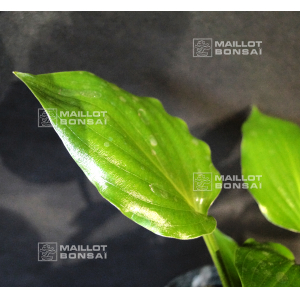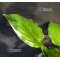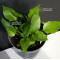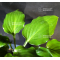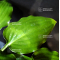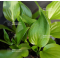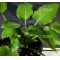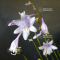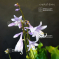ref. : 11099

10,00

Available quantity : 11Order

###### Description

Coming from a hybridization of Hosta Dixie chick.

Small hosta.

Dimensions of the leaves : about 8x5 cm.

Shiny dark green and softly corrugated leaves. This variety forms a pretty little and dense vegetal shrub.

Purple flowers from the middle of August to September.

Big rhizome delivered in a 1 liter plastic pot.

#hosta 4.5 #about 3.5 #dixie 3.5 #crystal 2.7 #leaves 2.6 #hostas 2.6 #from 2.4 #hybridization 2.3 #corrugated 2 #dimensions 2

Formule
(( ROUND((CHAR_LENGTH(b.article_nom)-CHAR_LENGTH(REPLACE(b.article_nom, 'hosta', '')))/LENGTH('hosta')) + ROUND((CHAR_LENGTH(b.article_description)-CHAR_LENGTH(REPLACE(b.article_description, 'hosta', '')))/LENGTH('hosta')) ) * 4.5) + (( ROUND((CHAR_LENGTH(b.article_nom)-CHAR_LENGTH(REPLACE(b.article_nom, 'about', '')))/LENGTH('about')) + ROUND((CHAR_LENGTH(b.article_description)-CHAR_LENGTH(REPLACE(b.article_description, 'about', '')))/LENGTH('about')) ) * 3.5) + (( ROUND((CHAR_LENGTH(b.article_nom)-CHAR_LENGTH(REPLACE(b.article_nom, 'dixie', '')))/LENGTH('dixie')) + ROUND((CHAR_LENGTH(b.article_description)-CHAR_LENGTH(REPLACE(b.article_description, 'dixie', '')))/LENGTH('dixie')) ) * 3.5) + (( ROUND((CHAR_LENGTH(b.article_nom)-CHAR_LENGTH(REPLACE(b.article_nom, 'crystal', '')))/LENGTH('crystal')) + ROUND((CHAR_LENGTH(b.article_description)-CHAR_LENGTH(REPLACE(b.article_description, 'crystal', '')))/LENGTH('crystal')) ) * 2.7) + (( ROUND((CHAR_LENGTH(b.article_nom)-CHAR_LENGTH(REPLACE(b.article_nom, 'leaves', '')))/LENGTH('leaves')) + ROUND((CHAR_LENGTH(b.article_description)-CHAR_LENGTH(REPLACE(b.article_description, 'leaves', '')))/LENGTH('leaves')) ) * 2.6) + (( ROUND((CHAR_LENGTH(b.article_nom)-CHAR_LENGTH(REPLACE(b.article_nom, 'from', '')))/LENGTH('from')) + ROUND((CHAR_LENGTH(b.article_description)-CHAR_LENGTH(REPLACE(b.article_description, 'from', '')))/LENGTH('from')) ) * 2.4) + (( ROUND((CHAR_LENGTH(b.article_nom)-CHAR_LENGTH(REPLACE(b.article_nom, 'hybridization', '')))/LENGTH('hybridization')) + ROUND((CHAR_LENGTH(b.article_description)-CHAR_LENGTH(REPLACE(b.article_description, 'hybridization', '')))/LENGTH('hybridization')) ) * 2.3) + (( ROUND((CHAR_LENGTH(b.article_nom)-CHAR_LENGTH(REPLACE(b.article_nom, 'corrugated', '')))/LENGTH('corrugated')) + ROUND((CHAR_LENGTH(b.article_description)-CHAR_LENGTH(REPLACE(b.article_description, 'corrugated', '')))/LENGTH('corrugated')) ) * 2) + (( ROUND((CHAR_LENGTH(b.article_nom)-CHAR_LENGTH(REPLACE(b.article_nom, 'dimensions', '')))/LENGTH('dimensions')) + ROUND((CHAR_LENGTH(b.article_description)-CHAR_LENGTH(REPLACE(b.article_description, 'dimensions', '')))/LENGTH('dimensions')) ) * 2) + (( ROUND((CHAR_LENGTH(b.article_nom)-CHAR_LENGTH(REPLACE(b.article_nom, 'delivered', '')))/LENGTH('delivered')) + ROUND((CHAR_LENGTH(b.article_description)-CHAR_LENGTH(REPLACE(b.article_description, 'delivered', '')))/LENGTH('delivered')) ) * 1.9)

## Secure payment## Delivery

Our logistic partners :04 74 55 23 48
Pépinière MAILLOT-BONSAÏ
Le Bois Frazy
01990 RELEVANT - FRANCE
on appointment